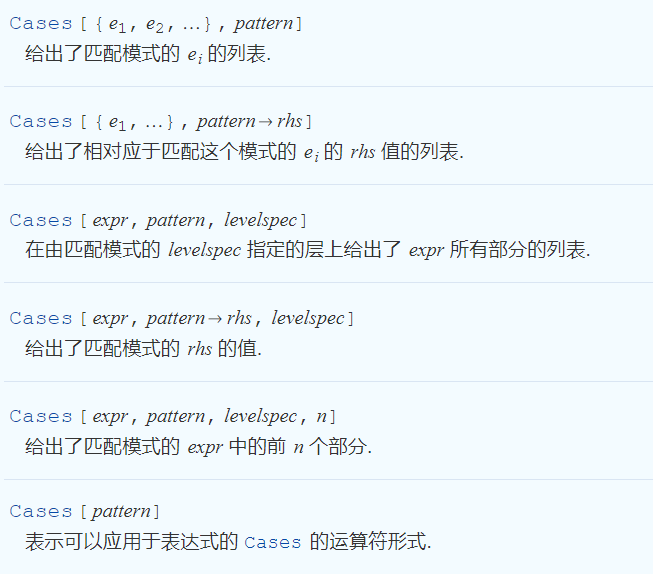### 规则

x^2+3/.x->2
out:7

#### 应用规则

ReplaceAll -- /.

x + 2 y /. {x -> y, y -> z}
out:
y + 2 z

ReplaceRepeated -- //.

ReplaceRepeated[1/(1 + x), x -> 1/(1 + x), MaxIterations -> 4]
out：
1/(1 + 1/(1 + 1/(1 + 1/(1 + 1/(1 + x)))))

#### mathematica中的规则

Solve[x^2 - 2 x == 0, x]
out：
{{x -> 0}, {x -> 2}}

x /. %

### 模式匹配

x^2 + x^3 /. a_^2 -> 2 a
out:
2 x + x^3

MatchQ[x^2, x^_Integer]
out: True
MatchQ[x^2, x^_Real]
out: False

#### 带条件的模式

MatchQ[5, _Integer?(# > 3 &)]
out:True

？的应用是很灵活的，并不一定要放到最后，我们需要对哪个"_"进行匹配，就需要放到其后面。比如：

MatchQ[x^2, _?(# == x &)^_]
out:True
MatchQ[x^2, _^_?(# == x &)]
out:False

### 模式在列表中的应用

Cases语法：Cases[{{1, 2}, {4, 3}, {5, 1}}, {_, _?(# > 1 &)}]
out:{{1, 2}, {4, 3}}  匹配第二项大于1的列表
Cases[{{1, 2}, {4, 3}, {5, 1}}, {a_, b_?(# > 1 &)} -> {a, x}]
out:{{1, x}, {4, x}}  匹配第二项大于1的列表，并将第二项替换为x

### 列表中的多个表达式

MatchQ[{x^2, x^3}, {_}]
out:False
MatchQ[{{x^2, x^3}}, {_}]
out:True

MatchQ[{x^2, x^3}, {__}]
out:True

MatchQ[{}, {_}]
out:False
MatchQ[{}, {__}]
out:False
MatchQ[{}, {___}]
out:True

Cases[Permutations[{1, 2, 3, 4}], {__, 2, ___, 4, ___}]
out:
{{1, 2, 3, 4}, {1, 2, 4, 3}, {1, 3, 2, 4}, {3, 1, 2, 4}, {3, 2, 1, 4}, {3, 2, 4, 1}}

2不在第一位，说明2前面要有一个或者多个表达式，可以用双下划线表示，而4在2的后面，说明2和4的中间有0、1或者多个表达式，可以用三个下划线表示，而4的后面也是这样，用三个下划线表示。

### 练习题

1、找到pattern

MatchQ[IntegerDigits, {___, 2, 0, 0, 7, ___}]
out:True

IntegerDigits /@ Prime /@ Range

2、使用Cases

Cases[IntegerDigits /@ Prime /@ Range, {___, 2, 0, 0, 7, ___}]

FromDigits /@ Cases[IntegerDigits /@ Prime /@ Range, {___, 2, 0, 0, 7, ___}]

## 发送评论编辑评论

|´・ω・)ノ
ヾ(≧∇≦*)ゝ
(☆ω☆)
（╯‵□′）╯︵┴─┴
￣﹃￣
(/ω＼)
∠( ᐛ 」∠)＿
(๑•̀ㅁ•́ฅ)
→_→
୧(๑•̀⌄•́๑)૭
٩(ˊᗜˋ*)و
(ノ°ο°)ノ
(´இ皿இ｀)
⌇●﹏●⌇
(ฅ´ωฅ)
(╯°A°)╯︵○○○
φ(￣∇￣o)
ヾ(´･ ･｀｡)ノ"
( ง ᵒ̌皿ᵒ̌)ง⁼³₌₃
(ó﹏ò｡)
Σ(っ °Д °;)っ
( ,,´･ω･)ﾉ"(´っω･｀｡)
╮(╯▽╰)╭
o(*////▽////*)q
＞﹏＜
( ๑´•ω•) "(ㆆᴗㆆ)
( ゜- ゜)つロ
_(:з」∠)_
（⌒▽⌒）
（￣▽￣）
(=・ω・=)
(*°▽°*)八(*°▽°*)♪
✿ヽ(°▽°)ノ✿
(¦3【▓▓】
눈_눈
(ಡωಡ)
_(≧∇≦」∠)_
━━━∑(ﾟ□ﾟ*川━
(｀・ω・´)
(￣3￣)
✧(≖ ◡ ≖✿)
(･∀･)
(〜￣△￣)〜
→_→
(°∀°)ﾉ
╮(￣▽￣)╭
( ´_ゝ｀)
←_←
(;¬_¬)
(ﾟДﾟ≡ﾟдﾟ)!?
( ´･･)ﾉ(._.)
Σ(ﾟдﾟ;)
Σ( ￣□￣||)<
(´；ω；)
（/TДT)/
(^・ω・^)
(｡･ω･｡)
(●￣(ｴ)￣●)
ε=ε=(ノ≧∇≦)ノ
(´･_･)
(-_-#)
（￣へ￣）
(￣ε(#￣) Σ
(╯°口°)╯(┴—┴
ヽ(Д´)ﾉ
("▔□▔)/
(º﹃º )
(๑>؂<๑）
｡ﾟ(ﾟ´Д｀)ﾟ｡
(∂ω∂)
(┯_┯)
(・ω< )★
( ๑ˊ•̥▵•)੭₎₎
¥ㄟ(´･ᴗ･)ノ¥
Σ_(꒪ཀ꒪」∠)_
٩(๛ ˘ ³˘)۶❤
(๑‾᷅^‾᷅๑)

Emoji

tv_小电视